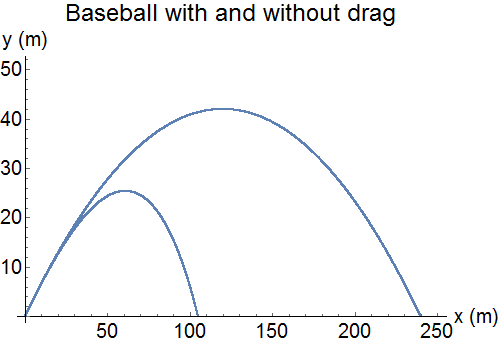## Mathematica tutorialsResults of a numeric integration to show the effects of air drag.

Computation plays an important role in physics. When teaching our first-year mechanics course, The Newtonian Synthesis (PHYS 123), I include a semester-long introduction to computational physics using the Mathematica software. The introduction consists of a series of tutorials (in the form of Mathematica notebooks) and follow-up assignments. These emphasize computer algebra, plotting, and numerically integrating equations of motion. As an introduction, many advanced features are left untouched. The assignments and tutorials are available below.